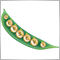CopyBuffer from another timeframe95

Hi all,

I'm new to MQL5 and I have a question about getting indicator data from another timeframe. Let's say I want to draw H1 Envelopes no matter what interval is displayed (M5, M15, M30 etc.). It means that for example on M15 the lines of the indicator should have the same value (or not?).

For now my code draws the envelope correctly only for H1.

onCalculate:

// CopyBuffer of Envelopes
int to_copy;

if(prev_calculated>rates_total || prev_calculated<=0)
to_copy=rates_total;
else
{
to_copy=rates_total-prev_calculated;
to_copy++;
}
CopyBuffer(envelopesHandle,0,0,to_copy,UpperBuffer);
CopyBuffer(envelopesHandle,1,0,to_copy,LowerBuffer);

and in onInit:

envelopesHandle=iEnvelopes(Symbol(),PERIOD_H1,ENVELOPES_ma_period,                             ENVELOPES_ma_shift,ENVELOPES_ma_method,ENVELOPES_applied_price,ENVELOPES_deviation);

As far as I remember in MQL4 there was a function to get bar number from another timeframe, and based on this number I could get the indicator value.

I will be grateful for any tips or links to any good articles.

Additional question is - looking through the forum I always find many snippets of code where people mention that we should check for errors etc. Yet in the documentation error checking is often omitted for the sake of simplicity of the example. For example CopyBuffer - for what errors should I check except for it returning -1?

Cheers6472

The values should be copied individually, using iBarShift().

//---
int envelopesHandle;
double Upper;
double Lower;
//+------------------------------------------------------------------+
//| Custom indicator initialization function                         |
//+------------------------------------------------------------------+
int OnInit()
{
//--- indicator buffers mapping
SetIndexBuffer(0,UpperBuffer,INDICATOR_DATA);
SetIndexBuffer(1,LowerBuffer,INDICATOR_DATA);
if((envelopesHandle=iEnvelopes(_Symbol,TimeFrame,ENVELOPES_ma_period,
ENVELOPES_ma_shift,ENVELOPES_ma_method,ENVELOPES_applied_price,ENVELOPES_deviation))==INVALID_HANDLE)
return(INIT_FAILED);
//---
return(INIT_SUCCEEDED);
}
//+------------------------------------------------------------------+
//| Custom indicator iteration function                              |
//+------------------------------------------------------------------+
int OnCalculate(const int rates_total,
const int prev_calculated,
const datetime &time[],
const double &open[],
const double &high[],
const double &low[],
const double &close[],
const long &tick_volume[],
const long &volume[],
{
//---
int begin=(prev_calculated>rates_total || prev_calculated<=0)?0:prev_calculated-1,shift=iBarShift(_Symbol,TimeFrame,time[rates_total-1]);
//---
if(BarsCalculated(envelopesHandle)<shift)
return(0);
//---
for(int i=begin;i<rates_total && !_StopFlag;i++)
{
shift=iBarShift(_Symbol,TimeFrame,time[i]);
if(CopyBuffer(envelopesHandle,0,shift,1,Upper)!=-1)
UpperBuffer[i]=Upper;
if(CopyBuffer(envelopesHandle,1,shift,1,Lower)!=-1)
LowerBuffer[i]=Lower;
}
//--- return value of prev_calculated for next call
return(rates_total);
}
//+------------------------------------------------------------------+95

Ernst Van Der Merwe:

The values should be copied individually, using iBarShift().

Thanks! Wow, that's pretty complicated for something I'd imagine should be easy...